## RADIO CALCULATIONSby Harry Lythall - SM0VPOHere are a few calculators for electronic/radio circuits. They are quite crude, but they work. Note that these formulas now accept "floating point" variables (an inductance value of 0.01 is a valid number).## Resistors In Series (or Capacitors in Parallel)## Resistors In Parallel (or Capacitors in Series)

Formula: Effective Resistance = (R1 x R2) / (R1 + R2)
(all units are the same, Ohms/Farads etc.)

 R1 R2 Effective Resistance## Reactance of a Capacitor

Formula: Reactance = 1 / (2 * Pi * F * C)

 Freq (Hz) [F] Cap (pF) [C] Reactance (Ohms)## Reactance of an Inductor

Formula: Reactance = 2 * Pi * F * L

 Freq (Hz) [F] Induct (uH) [L] Reactance (Ohms)## Basic frequency

Formula: Frequency (F) = 1 / (2 * Pi * Sqrt(LC))

 Capacitance (pF) [C] Inductance (uH) [L] Frequency (F)(Hz)## Single Layer Coil

 Formula: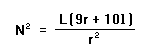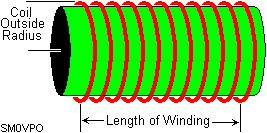Inductance (uH) [L] Length of Winding [l] Coil Outside Radius [r] All sizes in millimeters Turns required [N]## Multi-Layer Coil

 Formula: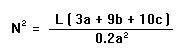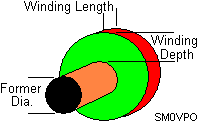Inductance (uH) [L] Winding depth+Form Dia [a] Winding Length [b] Winding Depth [c] Turns required (All sizes in millimeters)## Heatsink Evaluation

Calculates the effective temperature rise coefficient of a measured heatsink. Area is EXPOSED surface area of heatsink in square centimeters.

 Formula: Degrees Centigrade per Watt = 50 / Sqrt(Area sq-cm )

 Area (sq-cm) [A] Exposed areas only. Degrees C / Watt## Heatsink Calculation

Calculates heatsink surface area needed for a given heatsink temperature coefficient.

 Formula: Area Required (sq-cm) = Sq (50/C-watt)

 Degrees C / Watt Exposed areas only Area Required (sq-cm)## Attenuators - "T" and "H" Type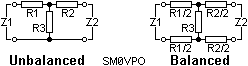Formulas: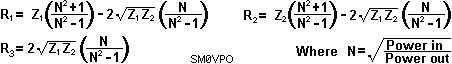The Input/Output powers may be in any electrical unit you prefer, eg: Meggawatts, Picowatts, Joules, Hamster-treadmill revolutions per hour etc.

 Enter Input Power Enter Output Power Enter Input Impedance Enter Output Impedance R1 value (for unbalanced) R2 value (for unbalanced) R1/2 value (for balanced) R2/2 value (for balanced) R3 value

Negative values of resistance occur when impossible values of input and output impedance and attenuation are entered. For example, it is not possible to drive a 50-ohm line from a 600-ohm line and have a voltage attenuation factor of less than 6.78## Attenuators - Square (or Box) Type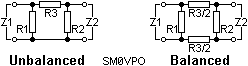Formulas: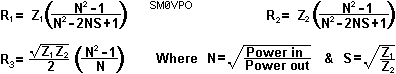The Input/Output powers may be in any electrical unit you prefer, eg: Meggawatts, Picowatts, Joules, Hamster-treadmill revolutions per hour, etc.

 Enter Input Power Enter Output Power Enter Input Impedance Enter Output Impedance R1 value R2 value R3 value (for unbalanced) R3/2 value (for balanced) SM0VPO

Again, negative values indicate an impossible attenuation situation.I will now research formulas for the calculation of alcoholic strength/sugar quantity for brewing as well as for calculating alcoholic content of the "mash" when the boiling temperature is known. If you have any information in these fields, or you think another aspect of radio/electronis would be usefull then please drop me a line. Very best regards from Harry - SM0VPO